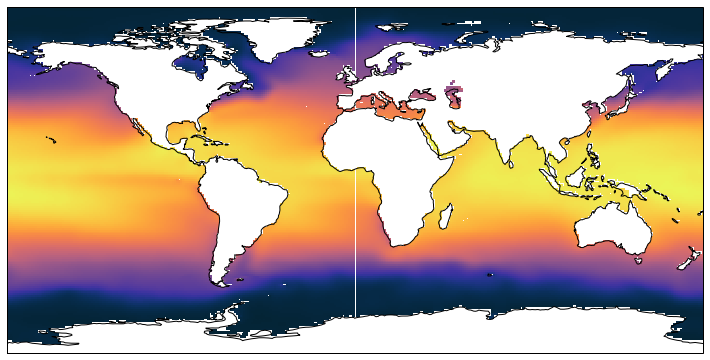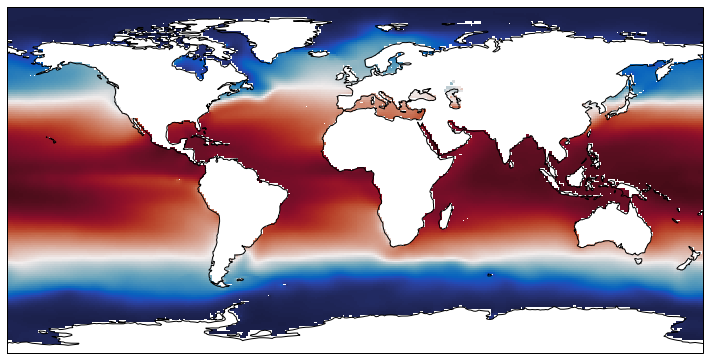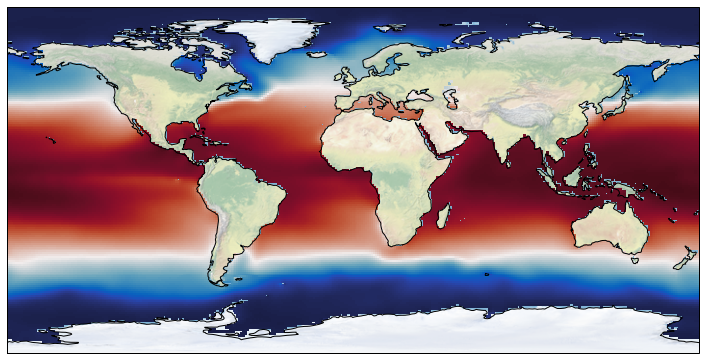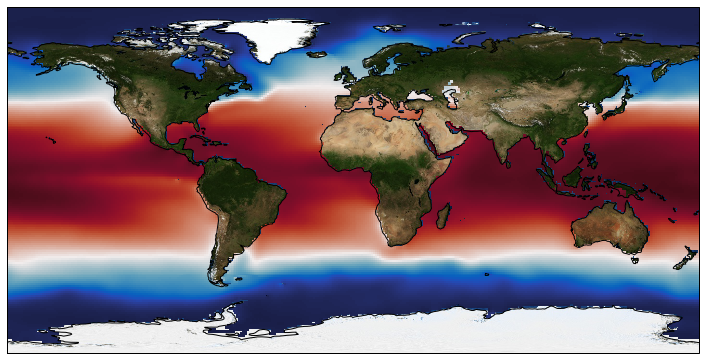# Background image in cartopy

Plot map of the temperature distribution in the ocean with some nice picture on the background.



Solution:

background_img function in cartopy 0.15

The cartopy is a great tool for creating maps in many ways more advanced than the usual workhorse for map creation in pyhton - the Basemap module. However I really missed one nice feature that Basemap have - easy way to add background image to the map. With the Basemap instance one can just write m.etopo() and get a relativelly nice map of the ETOPO topography overplayed or m.bluemarble() for the NASA's "Blue marble" image of the Earth . In the cartopy there is similar feature stock_img() but there is only one available image. In the newest version of cartopy developers add a background_img() method, that allows to add background images in a more convenient way. I will show you how to setup cartopy to use custom background images.

Imports

In :
from netCDF4 import Dataset, MFDataset, num2date
import matplotlib.pylab as plt
%matplotlib inline
import numpy as np
from matplotlib import cm
import cartopy.crs as ccrs
from cmocean import cm as cmo

import sys
import os


We will download World Ocean Atlas temperatures file to have something to plot.

In :
#!wget http://data.nodc.noaa.gov/thredds/fileServer/woa/WOA09/NetCDFdata/temperature_annual_1deg.nc


Open the file and read in coordinates

In :
flf = Dataset('./temperature_annual_1deg.nc')
lat = flf.variables['lat'][:]
lon = flf.variables['lon'][:]


Read in temperature from the first layer.

In :
temp = flf.variables['t_an'][0,0,:,:]


Make a basic cartopy plot:

In :
plt.figure(figsize=(13,6.2))

ax = plt.subplot(111, projection=ccrs.PlateCarree())

mm = ax.pcolormesh(lon,\
lat,\
temp,\
vmin=-2,\
vmax=30,\
transform=ccrs.PlateCarree(),cmap=cmo.thermal )
ax.coastlines();Ok, there is an ugly white line along 0 longitude, there is a simple way to fix it:

In :
temp_cyc, lon_cyc = add_cyclic_point(temp, coord=lon)

In :
plt.figure(figsize=(13,6.2))

ax = plt.subplot(111, projection=ccrs.PlateCarree())

mm = ax.pcolormesh(lon_cyc,\
lat,\
temp_cyc,\
vmin=-2,\
vmax=30,\
transform=ccrs.PlateCarree(),\
cmap=cmo.balance )
ax.coastlines();Looks fine. Now we will use default cartopy backgroung:

In :
plt.figure(figsize=(13,6.2))

ax = plt.subplot(111, projection=ccrs.PlateCarree())

mm = ax.pcolormesh(lon_cyc,\
lat,\
temp_cyc,\
vmin=-2,\
vmax=30, \
transform=ccrs.PlateCarree(),\
cmap=cmo.balance )
ax.coastlines();
ax.stock_img();Not that bad, but not exactly what I want.

Let's setup cartopy for use of the custom background. You have to have a folder that contain background images and a json file that describes images (see explination below). Then you have to create environment variable that contains the path to the folder:

In :
os.environ["CARTOPY_USER_BACKGROUNDS"] = "/home/magik/PYTHON/cartopy/BG/"


Now you can specify name of the image in it's resolution in the background_img() method:

In :
plt.figure(figsize=(13,6.2))

ax = plt.subplot(111, projection=ccrs.PlateCarree())
ax.background_img(name='BM', resolution='low')
mm = ax.pcolormesh(lon_cyc,\
lat,\
temp_cyc,\
vmin=-2,\
vmax=30,\
transform=ccrs.PlateCarree(),\
cmap=cmo.balance )
ax.coastlines(resolution='110m');And get a map with a nice background (Blue Marble in this case).

You can get a lot of nice map backgrounds from this NASA website https://neo.sci.gsfc.nasa.gov/.

The json file that you have to put in to the background folder should be called images.json and look like this:

{"__comment__": "JSON file specifying the image to use for a given type/name and
"BM": {
"__comment__": "Blue Marble Next Generation, July ",
"__source__": "https://neo.sci.gsfc.nasa.gov/view.php?datasetId=BlueMarbleNG
-TB",
"__projection__": "PlateCarree",
"low": "BM.jpeg",
"high": "BMNG_hirez.png"},

"pop": {
"__comment__": "Population density from Nasa earth observations website",
"__source__": "https://neo.sci.gsfc.nasa.gov/view.php?datasetId=SEDAC_POP",
"__projection__": "PlateCarree",
"high": "population.jpg"}
}

As you can see one can specify different "resolutions" for the same image name which will just point to different files.

That's it. Here is the video of ocean circulation that I have generated using this method. Below are just two more examples.

In :
plt.figure(figsize=(13,6.2))

ax = plt.subplot(111, projection=ccrs.Mercator(central_longitude=180, min_latitude=-30, max_latitude=67))
ax.background_img(name='BM', resolution='high')
ax.set_extent([30,-70,-30,70])
mm = ax.pcolormesh(lon,\
lat,\
temp,vmin=-2, vmax=30, transform=ccrs.PlateCarree(),cmap=cmo.balance )
ax.coastlines(resolution='10m');